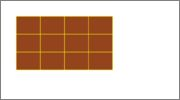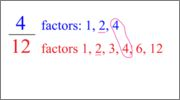# Definition of Simplest Form: Lesson for Kids

Instructor: Kathleen Laib

I have been a teacher for 10 years with eight of those years teaching 4th grade. I have a master's of Ed and an ELL Endorsement.

This lesson explains how to find the simplest form of a fraction. It not only builds the math skills necessary to find simplest form, but also puts simplest form into a real-life example.

Simplest form is really just about making a fraction simple, and what is simpler than smaller numbers? Finding the simplest form of a fraction means you are trying to make the numbers that make up the fraction--the numerator (top number) and denominator (bottom number)--as small as possible while still keeping the value of the fraction the same. In other words, you are finding the smallest possible equivalent fraction.

## Converting to Simplest Form

To find equivalent fractions, you need to multiply or divide by a fraction that is equivalent to 1. For example, 2/2, 3/3, 10/10, or 5/5 are all equivalent to 1, because you have all of the pieces you need to make the whole. Why do we need to use a fraction that's equivalent to 1? Because anytime you multiply or divide by 1, the amount doesn't change, so our new fraction will be equivalent to the original.

In finding the simplest form, our goal is to make both the numerator and denominator as small as possible. So, we want to divide by a fraction equivalent to 1. (Multiplying would make the original fraction larger, which is not what we want when simplifying.)

## Example Problem: Sharing Brownies

Let's say your mom makes a pan of brownies and cuts it into 12 equal pieces. She says you can have the ENTIRE pan of brownies--but you have to share it equally with two friends. She tricked you, but you still get a whole lot of brownies!You start handing out brownies among you and your two friends until all the brownies are gone. Each person ends up with 4 brownies. To turn this into a fraction, we would say that each person gets 4/12 of the pan of brownies, because there were 12 brownies in the whole pan and each person received 4 of those brownies.

### Finding Simplest Form

Now, let's find the simplest form of our fraction. To find simplest form, you need to divide by a fraction equivalent to 1. But which one? The first step is to make a factor list for the numerator and a factor list for the denominator. (Remember, factors of a given number are all the numbers you could multiply together to get that given number.) From those lists, find the greatest common factor.

For our brownie fraction, 4/12, you would list all the factors that make up 4 and 12, like this:To unlock this lesson you must be a Study.com Member.

### Register to view this lesson

Are you a student or a teacher?

#### See for yourself why 30 million people use Study.com

##### Become a Study.com member and start learning now.
Back
What teachers are saying about Study.com

### Earning College Credit

Did you know… We have over 200 college courses that prepare you to earn credit by exam that is accepted by over 1,500 colleges and universities. You can test out of the first two years of college and save thousands off your degree. Anyone can earn credit-by-exam regardless of age or education level.